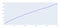# Just how many Prime Numbers are there? A simple pythonic approach

Mathematics seems to be fascinated by prime numbers and the number of prime numbers in particular. Prime numbers are said to be discovered by ancient greeks at around 300BC. Interestingly, one of the best methods for finding prime numbers was discovered by Greek known as Eratosthenes. Gauss, Legendre, and a myriad of other mathematicians later expanded these works.

A prime number theorem provides a way to approximate the number of primes less than or equal to a given number n. This value is called π(n), where π is the “prime counting function.” For example, π(10) = 4, since four primes are less than or equal to 10 (2, 3, 5, and 7). Similarly, π(100) = 25, since 25 of the first 100 integers are prime. Among the first 1,000 integers, there are 168 primes, so π(1,000) = 168, and so on. Note that as we considered the first 10, 100, and 1,000 integers, the percentage of primes went from 40% to 25% to 16.8%. These examples suggest, and the prime number theorem confirms, that the density of prime numbers at or below a given number decreases as the number gets larger.

But is there a way of determining all primes numbers up to a, let’s say a trillion. The prime number theorem offers an approximation to solve this computationally vast problem.
Basically, as per theorem π(n) or the number of primes occurring, n is equal to n * ln(n), where ln is the natural logarithm. This is because the ratio of π(n) to n/ ln(n) approaches what is known as an asymptotic value near infinity, which means at large values of n, it becomes constant. This seems counter-intuitive as the gaps between prime numbers increase almost exponentially, but we will see later on that even with a simple function, this relationship seems true.

We will use simple python functions and computations to find work on the Prime Number Theorem instead of using complicated mathematics.
Let’s start with the most basic algorithm for generating Prime numbers, the legendary “Sieve of Eratosthenes.”

The algorithm’s gist goes that we create a list of prime number candidates till the maximum number specified. Using repeated filters or sieves, which are essentially the first few prime numbers, i.e., 2,3,5, we remove the divisible numbers by these first few primes. So, essentially the sieve is the divisibility test of a number with a known prime.

Let's start with build a basic sieve function.

`import mathfrom typing import List, Callableimport numpy as npimport matplotlib.pyplot as pltimport timedef SieveOfEratosthenes(N:int) -> List[int]:    #N is the no. till prime    prime_no_results_array = []        prime_numbers:List[int] = [True for i in range(N+1)]        __STARTING_PRIME_SIEVE:int = 2    prime_no_sieve:int = __STARTING_PRIME_SIEVE    #Now the algo says that we must remove each element divisible by a sieve    while (prime_no_sieve ** 2 <= N):         #Check if prime_no_sieve is divisible        if (prime_numbers[prime_no_sieve] == True):                         for i in range(prime_no_sieve**2, N+1, prime_no_sieve):                prime_numbers[i] = False        prime_no_sieve += 1     # Print all prime numbers    for no in range(2, N+1):        if prime_numbers[no]:            prime_no_results_array.append(no)    return prime_no_results_array`

The above code, although workable, is a little slow. Let replace some parts using the NumPy library

`def SieveOfEratosthenesWithNumpy(N:int) -> np.array:       prime_numbers:List[int] = np.ones(N,dtype=bool)    prime_numbers = prime_numbers = False        for sieve_no in range(2,N):        if prime_numbers[sieve_no]:                        prime_numbers[sieve_no*sieve_no::sieve_no] = False    return np.flatnonzero(prime_numbers)`

Let's write a timer function to check out function execution timelines

`def time_my_primes(func:Callable[[int],List],no_array:List[int]) -> List[float]:    __temp = []    for i in no_array:        t1 = time.time()        func(i)        t2= time.time()        #where time is stores in milliseconds        __temp.append((t2-t1)*1000)                    plt.plot(np.array(no_array),np.array(__temp))    return __temp`

The sieve-based or deterministic method however fails to generate truly large prime numbers required for computing purposes. For RSA prime number generation we require probability methods such as the Miller-Rabin test with some acceptable level of error such as 1/2e128. These tests will essentially give you a probability that the number is prime instead of finding the exact prime for a given N.

We can use the python Crypto library to generate prime of N bits, but since it uses probabilistic methods it is not suitable for our analysis.

`from Crypto.Util import number def SieveOfEratosthenesRandom(N):        result = set()    #adding Randomness will be inaccurate for lower primes    for i in range(1,N):        result.add(number.getPrime(i))    return list(result)`

Let's build a simple computation loop for checking the hypothesis of the theorem as discussed above.

`import plotly.express as px def Pi(No:int,func:Callable[[int],list]) -> int:    return len(func(No))def ApproximationFunc(No:int) -> float:    return No/math.log(No,math.exp(1))#write our ploting functiondef plotter(N:int,interval:int=100) -> None:    loader_Pi = []    loader_approx = []             for i in range(N,N*interval):        loader_Pi.append(Pi(i,SieveOfEratosthenesWithNumpy))        loader_approx.append(ApproximationFunc(i))    import plotly.graph_objects as gofig = go.Figure()    fig.add_trace(go.Scatter(x=np.arange(N,N*interval), y=loader_Pi,                    mode='lines',                    name='Pi(N)'))    fig.add_trace(go.Scatter(x=np.arange(N,N*interval), y=loader_approx,                    mode='lines+markers',                    name='LogApproximation'))    fig2 = go.Figure()    fig2.add_trace(go.Scatter(x=np.arange(N,N*interval), y=np.array(loader_Pi)-np.array(loader_approx),                    mode='lines',                    name='Pi(N)'))fig.show()fig2.show()`

The approximation much closely for the first 25000 natural numbers

We can see from the first experiments that the ratio of prime numbers to natural numbers falls drastically as N increases, so each corresponding interval will produce fewer prime numbers. Let check this by creating a prime gap function

`def plotterForGaps(N:int,interval:int=5) -> None:    loader = []                 for i in range(N,N*interval):        loader.append(np.log(number.getPrime(i) - number.getPrime(i-1)))            import plotly.graph_objects as go    fig = go.Figure()    fig.add_trace(go.Scatter(x=np.arange(N,N*interval), y=loader,                    mode='lines',                    name='log of Gaps'))    fig.show()`

So in the above figure, the x-axis is 2 N bit based and the y-axis is the log of difference between two primes

What about the error of π(n) and its approximate functionThe error shows a near straight line on log scale

So how many prime numbers are there?

N / Ln(N) seems to be a good approximation as shown above. Hence for the 1 trillion intervals, there must be at least 3.6% of primes. For the 1e20 interval, there must be at least around 2.2% prime numbers in the interval.